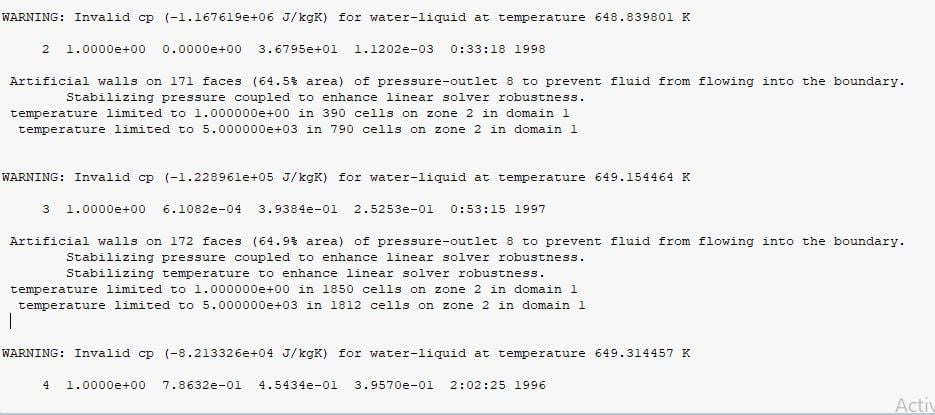## Fluids

•atulsbhat
Subscriber

PROBLEM EXPLANATION : A NATURAL CONVECTION PROBLEM

Geometry : 2D Rectangular Flat Plate

SOLVER: PRESSURE BASED,STEADY STATE , LAMINAR, SINGLE PHASE AND WITH ENERGY MODEL

GRAVITY: Y= -9.81

MATERIAL PROPERTIES: UDF (CELL BASED)

BOUNDARY CONDITIONS:

ISO (WALL) = 649.16 K (Near Critical Region TEMP) {Non LINEAR}

VELOCITY INLET= 0.0001 m/sec and 643.16 K

PRESSURE OUTLET: 22500000 (No Back Flow)

Method :COUPLED AND ALL RELAXATION FACTORS UNDER 0.8

UDF IS ATTACHED in the link above(NAMED PRO)

ERRORS WHICH I GOT; INVALID CP (I SET TEMP BOUNDS RIGHT)Regards ATUL S

•DrAmine
Ansys Employee
As ANSYS employee I do ignore attachments and links to Deive ObeDrive etc...

Paste your UDF here. Just for superficial verification as we do not debug UDFs
•atulsbhat
Subscriber

#include "udf.h"

DEFINE_PROPERTY(cell_density, c, t)

double T2 = C_T(c, t);

double rho;

if (635.00< T2 <=647.65)

{

rho = (-1.80375321498E-008*pow(T2,3)+4.37725881273E-003*pow(T2,2)-5.72870568035E+000*T2+1.87919262419E+003)/(5.73224251694E-009*pow(T2,3)-5.29420035132E-006*pow(T2,2)-5.19145559843E-004*T2+1);

}

else if (647.65< T2 <=648.70)

{

rho = (3.17383063793E-005*pow(T2,3)-4.67841673791E-002*pow(T2,2)+2.04434456269E+001*T2-2.23825052795E+003)/(6.58499450671E-009*pow(T2,3)-6.85372588417E-006*pow(T2,2)+1.33555488262E-004*T2+1);

}

else if (648.70< T2 <=649.00)

{

rho-4.34313022431E-005*pow(T2,3)+3.32633967077E-002*pow(T2,2)+1.16448492280E+001*T2-9.69578668950E+003)/(-8.21772033015E-009*pow(T2,3)+1.25803346920E-005*pow(T2,2)-6.24451347023E-003*T2+1);

}

else if (649.00< T2 <=660.00)

{

rho = (-9.94061577245E-007*pow(T2,3)+2.29499012449E-003*pow(T2,2)-1.71573797723E+000*T2+4.18604178695E+002)/(1.54441783415E-009*pow(T2,3)+4.23867511741E-007*pow(T2,2)-2.46639558372E-003*T2+1);

}

else

{

rho = 145;

}

return rho;

}

DEFINE_SPECIFIC_HEAT(cell_SpecificHeat, T, Tref, h, yi)

{

double cp;

if (635.00< T <=648.00)

{

cp=(-1.22158459347E-003*pow(T,3)+2.17569323612E+000*pow(T,2)-1.29281382835E+003*T+2.56632684171E+005)/(-1.54130619189E-003*T+1);

}

else if (648.00< T <=648.73)

{

cp=(3.55323794766E-004*pow(T,3)-4.48432744279E-001*pow(T,2)+ 1.33037573218E+002*T+5.40783052792E+003)/(4.28000889300E-012*pow(T,4)+2.41784831000E-010*pow(T,3)-3.34155028245E-006*pow(T,2)-6.43985888337E-004*T+1);

}

else if (648.73< T <=649.50)

{

cp=(-5.18823303098E-008*pow(T,4)+8.61428212182E-004*pow(T,3)-1.43401674862E+000*pow(T,2)+8.29938510799E+002*T-1.60895776843E+005)/(4.17611916514E-009*pow(T,3)-3.04175710540E-006*pow(T,2)-1.32571651328E-003*T+1);

}

else if (649.50< T <=660.00)

{

cp=(3.63087828676E-007*pow(T,4)-5.05376552107E-004*pow(T,3)+7.74302775445E-002*pow(T,2)+1.42466716874E+002*T-5.13360638841E+004)/(6.70506677622E-009*pow(T,3)-6.23021496014E-006*pow(T,2)-3.21570998725E-004*T+1);

}

else

{

cp = 10000;

}

*h = cp*(T-Tref);

return cp;

}

•atulsbhat
Subscriber

Continuation.....

DEFINE_PROPERTY(cell_ktc, c, t)

double T3 = C_T(c, t);

double ktc;

if (635.00< T3 <=647.95)

{

ktc = (9.67327651787E-010*pow(T3,3)+ 1.07198980062E-006*pow(T3,2)-2.65628458181E-003*T3+1.00798585123E+000)/(5.00871983799E-009*pow(T3,3)-4.30553217646E-006*pow(T3,2)-8.56213098793E-004*T3+1);

}

else if (647.95< T3 <=648.73)

{

ktc = (-3.15665107745E-011*pow(T3,4)+5.74316328609E-008*pow(T3,3)-8.00570356905E-005*pow(T3,2)+6.58380991564E-002*T3-1.91080817698E+001)/(-1.88933190656E-007*pow(T3,3)+2.47518298176E-004*pow(T3,2)-8.26013714844E-002*T3+1);

}

else if (648.73< T3 <=649.10)

{

ktc = (9.21882104159E-010*pow(T3,3)+9.66700804229E-007*pow(T3,2)-2.41830902924E-003*T3+9.10302469777E-001)/(6.85095548536E-009*pow(T3,3)-6.51237771764E-006*pow(T3,2)-1.99926781030E-004*T3+1);

}

else if (649.10< T3 <=649.90)

{

ktc = (1.55340145958E-009*pow(T3,3)-2.08750317819E-006*pow(T3,2)+7.41797773475E-004*T3-2.68104657893E-002)/(3.01622383420E-011*pow(T3,3)+2.30136429280E-006*pow(T3,2)-3.04713864718E-003*T3+1);

}

else if (649.90< T3 <=660.00)

{

ktc = (1.57649522842E-010*pow(T3,3)+2.60321568704E-007*pow(T3,2)-5.21436191042E-004*T3+1.85693253101E-001)/(6.17253403657E-009*pow(T3,3)-5.52538004836E-006*pow(T3,2)-5.54654898842E-004*T3+1);

}

else

{

ktc = 0.15000;

}

return ktc;

}

DEFINE_PROPERTY(cell_viscosity, c, t)

double T4 = C_T(c, t);

double mu_lam;

if (635.00< T4 <=647.20)

{

mu_lam = (9.89197719095E-015*pow(T4,4)-1.71009457131E-011*pow(T4,3)+9.33538304205E-009*pow(T4,2)-1.41644707543E-006*T4-9.28741686026E-005)/(-1.53533206630E-003*T4+1);

}

else if (647.20< T4 <=648.60)

{

mu_lam = (3.99280946668E-013*pow(T4,3)-1.98818294190E-010*pow(T4,2)-3.35610542542E-007*T4+1.92473554834E-004)/(-1.53822789587E-003*T4+1);

}

else if (648.60< T4 <=648.80)

{

mu_lam = (4.49491045673E-011*pow(T4,2)-5.82458544750E-008*T4+1.88689994165E-005)/(2.09494866252E-009*pow(T4,3)-3.38328960437E-007*pow(T4,2)-2.20364870424E-003*T4+1);

}

else if (648.80< T4 <=649.10)

{

mu_lam = (-3.70888390134E-016*pow(T4,4)-2.66822899344E-013*pow(T4,3)+2.04526782970E-010*pow(T4,2)+4.75118757913E-007*T4-2.55763296559E-004)/(2.20110380648E-009*pow(T4,3)-6.08541480188E-007*pow(T4,2)-2.07309915781E-003*T4+1);

}

else if (649.10< T4 <=660.00)

{

mu_lam = (-1.05232848074E-014*pow(T4,4)+1.59353912056E-011*pow(T4,3)-4.11811500648E-009*pow(T4,2)-3.32793940923E-006*T4+1.40518231742E-003)/(-1.54325740965E-003*T4+1);

}

else

{

mu_lam = 0.000027000;

}

return mu_lam;

}

•DrAmine
Ansys Employee

Can you then start debugging a bit by setting some properties constant and focusing on the one causing the issue?

•ani4377
Subscriber

Dear Atul,

Did you find the reason for error?.

Regards

Anirudh

•ani4377
Subscriber

Can you then start debugging a bit by setting some properties constant and focusing on the one causing the issue?

Sir I am facing the same kind of error.

Can you please take a look at my post.

Thank you.

Anirudh

•atulsbhat
Subscriber

Hello

I did not find any error but the property plot at end of my results, Showed me different set of properties, Well I cross checked all polynomials again , The polynomials seemed correct but properties fed into fluent didnt match with the Polynomial properties..

Regards

•Rob
Ansys Employee

If you're using polynomials with that many significant figures it's very likely that single/double precision and rounding error will generate significant errors.  Post an image of what the curve should look like.

•atulsbhat
Subscriber
•Rob
Ansys Employee

And if you overlay polynomial on top?